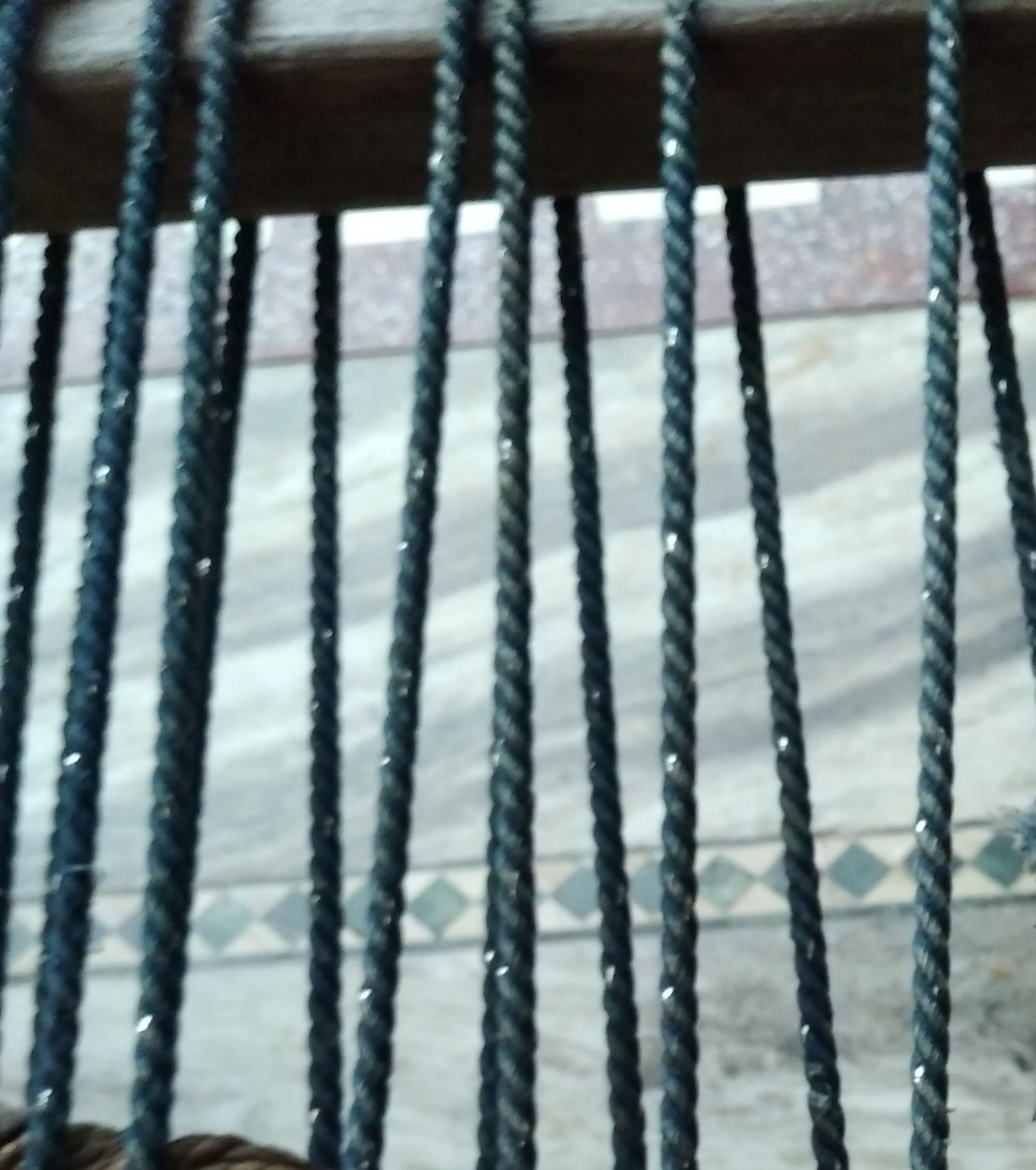Symbol
Problem$2.$ Evaluate $\int ^{3}|x^{2}-2x|$ $dx$ $1$ $3.$ If the function $f\left(x\right)=\left(\dfrac {x^{2-1}} {x-1}$ $k$ when $x≠1$ when $x=1$ is given to be continuous at $x=1,$ then the value of $k$ is
Physics
Search count: 1,541
SolutionQanda teacher - shivasir
Ring rolling is a hot forming process that produces seamless rings varying in size from a few inches in diameter, and weighing less than one pound, to over 25 feet in diameter and face heights approaching 10 feet. The process and equipment are similar in principle to rolling mills used for plate.